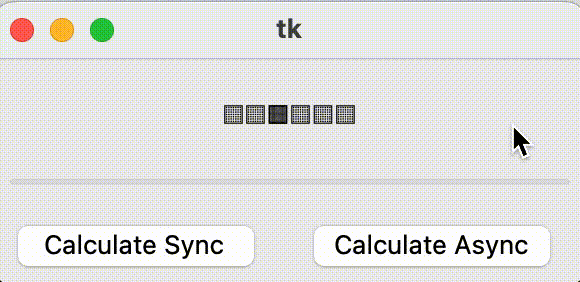GUI apps have an event loop. Asyncio has an event loop. So what if you want to create a Tkinter app that uses asyncio? Can you merge both event loops? With a simple trick you can keep the UI responsive by calling Tkinters update method from an infinite loop in an awaitable function.

# Python asyncio with Tkinter.

Look at this function:

```def calculate_sync(self):
max = 3000000
for i in range(1, max):
self.progressbar["value"] = i / max * 100```

When this function is executed synchronously, the GUI freezes.

## Blocking and freezing the UIBy clicking the 'Calculate Sync' button, the function is called and the GUI freezes. After a few seconds, the progress bar is 100% full and the GUI gets responsive again.

But what you want, is that the function runs while updating the UI.

## Non-blockingThis time, the 'Calculate Async' button is clicked and the function executes asynchronously and the GUI stays responsive.

## Solution

The following code creates an app that creates a window. The window gets the asyncio event loop.

__init__ creates a window and the show function creates an infinite loop that calls self.root.update(). update() keeps the GUI event loop running.

```import tkinter as tk
from tkinter import ttk
import asyncio

class App:
async def exec(self):
self.window = Window(asyncio.get_event_loop())
await self.window.show();

class Window(tk.Tk):
def __init__(self, loop):
self.loop = loop
self.root = tk.Tk()
self.animation = "░▒▒▒▒▒"
self.label = tk.Label(text="")
self.progressbar = ttk.Progressbar(length=280)
button_block = tk.Button(text="Calculate Sync", width=10, command=self.calculate_sync)
button_non_block = tk.Button(text="Calculate Async", width=10, command=lambda: self.loop.create_task(self.calculate_async()))

async def show(self):
while True:
self.label["text"] = self.animation
self.animation = self.animation[1:] + self.animation
self.root.update()
await asyncio.sleep(.1)

def calculate_sync(self):
max = 3000000
for i in range(1, max):
self.progressbar["value"] = i / max * 100

async def calculate_async(self):
max = 3000000
for i in range(1, max):
self.progressbar["value"] = i / max * 100
if i % 1000 == 0:
await asyncio.sleep(0)

asyncio.run(App().exec())```

There is no need to install any third party library. Copy and paste this code and run it with Python to test it yourself.

Written by Loek van den Ouweland on 2022-04-08.
Questions regarding this artice? You can send them to the address below.